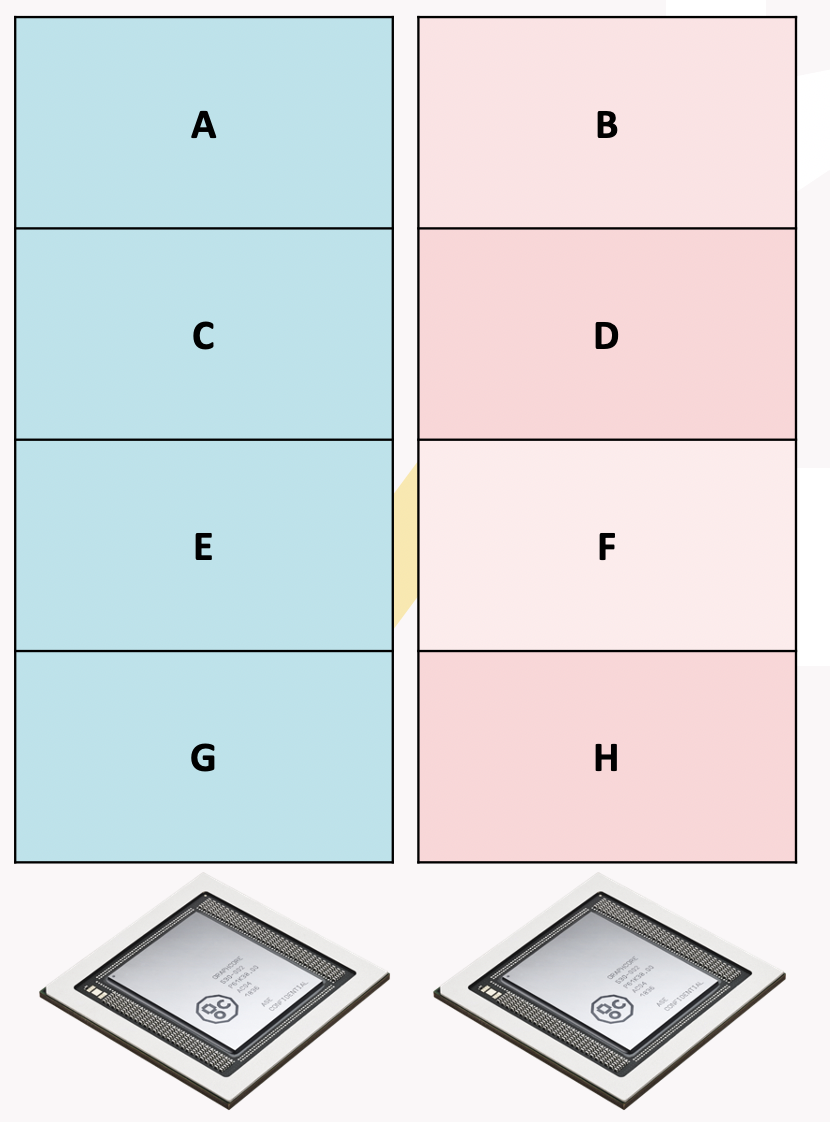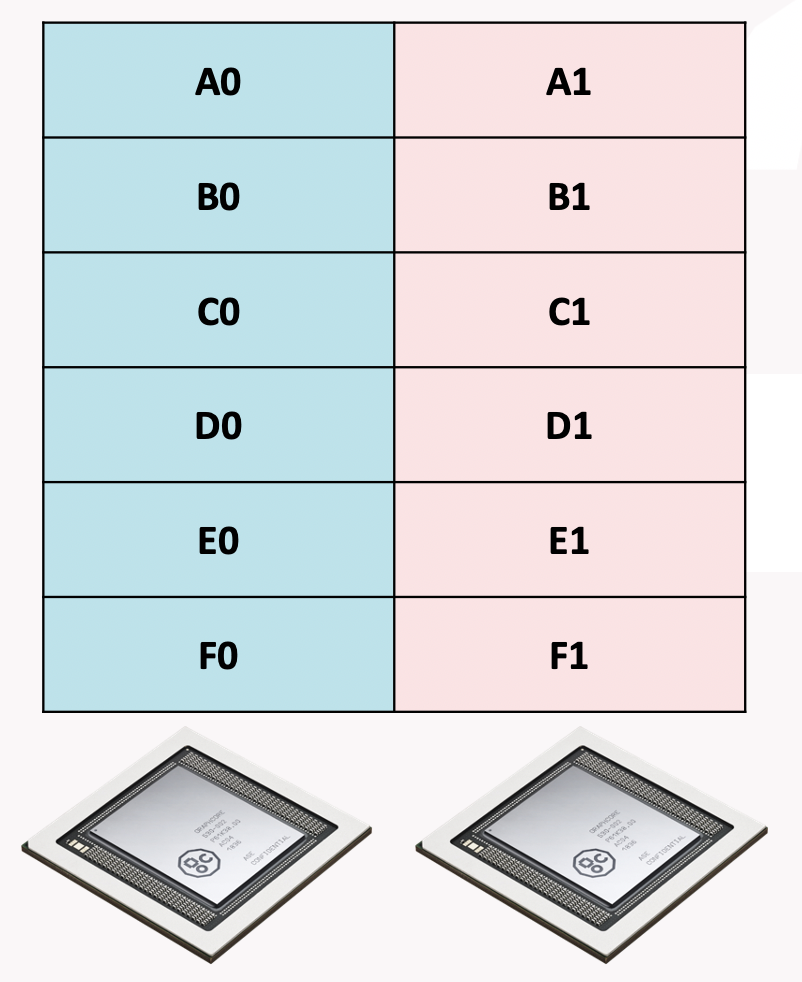# 15. IPU host embeddings¶

An embedding table is a table in a model or compute graph that supports a lookup operation. For more details see the TensorFlow documentation on tf.nn.embedding_lookup.

On the IPU, large embeddings can be stored in host memory with the CPU performing the lookup operations (and update operations during training) in conjunction with the IPU. This functionality supports both inference and training.

During execution the IPU will synchronize with the host and send indices (and possibly update values) to the host CPU. The CPU will then perform the lookup or update operation in a callback operation before returning the result to the IPU. The IPU will then carry on execution.

Applications access this functionality through the `tensorflow.python.ipu.embedding_ops.HostEmbedding` class and the `tensorflow.python.ipu.embedding_ops.create_host_embedding()` helper function. Optimisation of the host embedding is described in the `tensorflow.python.ipu.embedding_ops.HostEmbeddingOptimizerSpec` class, which currently only supports SGD with a constant learning rate.

Note

IPU host embeddings are not recommended for use in pipelines and will likely decrease the pipeline’s parallel efficiency.

## 15.1. Usage¶

IPU host embeddings rely on instances of the `HostEmbedding` class to coordinate the communication between the host and device. This object is created with a call to `tensorflow.python.ipu.embedding_ops.create_host_embedding()`. The created object is then passed to the user model where the `tensorflow.python.ipu.embedding_ops.HostEmbedding.lookup()` method can be called with a similar API to `tf.nn.embedding_lookup`.

Once the IPU host embedding has been created and used within the model, the object must be “registered” with the session using the context manager created by (`tensorflow.python.ipu.embedding_ops.HostEmbedding.register()`). If TensorFlow session is not called within this context, TensorFlow will not configure the underlying Poplar engine correctly and the model execution will fail.

## 15.2. Example¶

 ``` 1 2 3 4 5 6 7 8 9 10 11 12 13 14 15 16 17 18 19 20 21 22 23 24 25 26 27 28 29 30 31 32 33 34 35 36 37 38 39 40 41 42 43 44 45 46 47 48 49 50 51 52 53 54 55 56 57 58 59 60 61 62 63 64 65 66 67 68 69 70 71 72 73 74 75 76 77 78 79 80 81 82 83 84 85 86 87 88 89 90 91 92 93 94 95 96 97 98 99 100 101 102 103 104 105 106 107 108 109 110 111 112 113 114 115 116 117 118 119 120 121 122 123``` ```import numpy as np import tensorflow as tf from tensorflow.python.ipu import embedding_ops from tensorflow.python.ipu import ipu_compiler from tensorflow.python.ipu import ipu_infeed_queue from tensorflow.python.ipu import loops from tensorflow.python.ipu import cross_replica_optimizer from tensorflow.python.ipu import scopes from tensorflow.python.ipu import rnn_ops from tensorflow.python import ipu from tensorflow.python import keras path_to_file = keras.utils.get_file( 'shakespeare.txt', 'https://storage.googleapis.com/download.tensorflow.org/data/shakespeare.txt' ) # Read, then decode for py2 compat. text = open(path_to_file, 'rb').read().decode(encoding='utf-8') # The unique characters in the file vocab = sorted(set(text)) # Creating a mapping from unique characters to indices char2idx = {u: i for i, u in enumerate(vocab)} idx2char = np.array(vocab) text_as_int = np.array([char2idx[c] for c in text]).astype(np.int32) sequence_length = 100 batch_size = 16 replication_factor = 2 # Create training examples / targets ds = tf.data.Dataset.from_tensor_slices(text_as_int) ds = ds.batch(sequence_length, drop_remainder=True) ds = ds.shuffle(batch_size * batch_size) ds = ds.batch(batch_size, drop_remainder=True) ds = ds.repeat() # The host side queues infeed_queue = ipu_infeed_queue.IPUInfeedQueue(ds) # Set the learning rate lr = 0.0001 # Create a momentum optimiser for replication optimizer = cross_replica_optimizer.CrossReplicaOptimizer( tf.train.MomentumOptimizer(lr, 0.99)) # Create a host embedding object embedding = embedding_ops.create_host_embedding( "char_embedding", shape=[256, 256], dtype=tf.float32, partition_strategy="TOKEN", optimizer_spec=embedding_ops.HostEmbeddingOptimizerSpec(lr)) # PopnnGRU is time-major def gru(partials): gru_ = rnn_ops.PopnnGRU(256) partial_t = tf.transpose(partials, [1, 0, 2]) gru_outputs_t, _ = gru_(partial_t) return tf.transpose(gru_outputs_t, [1, 0, 2]) # The main model def model(sequence): # Perform a lookup on the embedding partial = embedding.lookup(sequence) partial = gru(partial) partial = tf.reshape(partial, [partial.shape, -1]) partial = tf.layers.dense(partial, 256) return tf.nn.softmax(partial) # Compute the loss for a given batch of examples def evaluation(sequence): # Use the last element of the sequence as the label to predict label = tf.slice(sequence, [0, sequence_length - 1], [-1, 1]) sequence = tf.slice(sequence, [0, 0], [-1, sequence_length - 1]) logits = model(sequence) return keras.losses.sparse_categorical_crossentropy(label, logits) # Minimise the loss def training(loss, sequence): loss = evaluation(sequence) mean_loss = tf.math.reduce_mean(loss) train = optimizer.minimize(loss) return mean_loss, train num_iterations = 1000 # Loop over our infeed queue, training the model def my_net(): loss = tf.constant(0.0, shape=[]) r = loops.repeat(num_iterations, training, [loss], infeed_queue) return r # Compile the model with scopes.ipu_scope('/device:IPU:0'): run_loop = ipu_compiler.compile(my_net, inputs=[]) # Configure the hardware config = ipu.config.IPUConfig() config.auto_select_ipus = replication_factor config.configure_ipu_system() with tf.Session() as sess: sess.run(tf.global_variables_initializer()) sess.run(infeed_queue.initializer) # Train the model for some iterations with embedding.register(sess): for i in range(25): l = sess.run(run_loop) print("Step " + str(i) + ", loss = " + str(l)) ```

## 15.3. Experimental functionality: IPU embeddings in remote buffers¶

As an alternative to host embeddings, there is experimental functionality to store embedding tables in remote buffer memory (i.e. off-chip memory directly accessed by the IPU). In this case the IPU performs the lookup/update operations directly on the remote buffer memory and the host CPU is not involved.

Setting the experimental.enable_remote_buffer_embedding option on an `IPUConfig` to `True` (defaults to `False`) and then configuring the IPU system with that config will cause the IPU host embedding implementation to globally use remote buffer embeddings instead.

Note

This option is experimental, and may be changed or removed in future releases.

### 15.3.1. Partitioning strategies¶

When using IPU embeddings in remote buffers together with data-parallel replication, the embedding table is not duplicated for each replica. Instead, a single copy of the table is shared between replicas to make the most of available memory. However, each replica only has access to a distinct memory space so the table is partitioned into chunks between the replicas (this holds even on hardware platforms like the DSS-8440 server where IPUs share physical external memory).

The way the table is split between the memory attached to each replica is determined by the partitioning strategy. Two partitioning strategies are available. These are the token strategy and the encoding strategy. Each has trade-offs and the choice of strategy will depend on the application. The partition strategy is set via the `partition_strategy` keyword argument of `tensorflow.python.ipu.embedding_ops.create_host_embedding()`.

#### Token strategy¶

The token strategy partitions the embedding on the token axis. There will be `ceil(t/r)` whole tokens on each replica, where `t` is the token count and `r` is the replica count.When this strategy is used, cross-replica operations are required to allow each replica to perform a lookup or update across the whole table (each replica’s portion of the whole embedding table is private to that replica). Below is the pseudo-code, with explicit types and static shapes, for how this is implemented:

 ``` 1 2 3 4 5 6 7 8 9 10 11 12 13 14 15 16 17 18 19 20 21 22 23 24 25 26 27 28 29 30 31``` ```// Pseudo-code assuming we have table size `t`, and replica count `r`. f16[14, 64] global_lookup( local_table : f16[ceil(t/r), 64] global_indices : i32 ): // The unique replica ID for "this" replica. replica_id = i32[] get-replica-id // Distribute the indices to all devices. indices = all-gather(indices) : i32[r, 14] // Scale the indices down by the replication factor. Indices not meant for // this replica will map to a valid, but incorrect index. local_indices = indices / r : i32[r, 14] // Gather on the local embedding region. result = lookup(embedding, indices) : f16[r, 14, 64] // The mask of which indices are valid. mask = (indices % r) == replica_id : bool[r, 14] // Zero out the invalid regions of the result result = select(result, 0, mask) : f16[r, 14, 64] // Reduce scatter sum the masked result tensor. The zeroed regions of the // result tensor ensure that invalid values are ignore and each replica has // the correct result. result = reduce-scatter-sum(result) : f16[1, 14, 64] // Reshape to the expected shape return reshape(result), shape=[14, 64] : f16[14, 64] ```

#### Encoding strategy¶

The encoding strategy will partition the embedding on the encoding axis. There will be `ceil(1/r)` of every tokens on each replica, where `r` is the replica count. This means for a given token every replica will store `ceil(e/r)` elements, where `e` is the element count for a single token.When this strategy is used, cross-replica operations are required to allow each replica to perform a lookup or update across the whole table (each replica’s portion of the whole embedding table is private to that replica). Below is the pseudo-code, with explicit types and static shapes, for how this is implemented:

 ``` 1 2 3 4 5 6 7 8 9 10 11 12 13 14 15 16 17 18 19 20 21 22 23 24 25``` ```// Pseudo-code assuming we have table size `t`, replica count `r`, and // encoding size `e`. f16[14, e] global_lookup( local_table : f16[t, ceil(e/r)] global_indices : i32 ): // Distribute the indices to all devices indices = all-gather(global_indices) : i32[r, 14] // Gather on the local embedding result = lookup(local_embedding, indices) : f16[r, 14, ceil(e/r)] // Communicate the relevant parts of the embedding to their respective // replicas. This distributes the ith slice in the outermost dimension to // ith replica. result = all-to-all(result, slice_dim=2, concat_dim=3) : f16[r, 14, ceil(e/r)] // Transpose the dimensions back into the correct order. result = transpose(result), permutation=[1, 0, 2] : f16[14, r, ceil(e/r)] // Flatten the innermost dimensions result = flatten(result), begin=1, end=2 : f16[14, r*ceil(e/r)] // Slice off the excess padding on the encoding return slice(result), dim=1, begin=0, end=e : f16[14, e] ```

#### Choosing a strategy for your application¶

The choice of partitioning strategy is application dependent and the best way to determine the best strategy is to profile multiple strategies.

As a general rule, the token strategy is used when the encoding is much smaller than the token count. An example application for this would be language models where the vocabulary size is much larger than the encoding.

Conversely, the encoding strategy is used when the token count is small and the encoding is large enough to be split. This avoids a large amount of very small communication. An example application for this would be game playing models, where a small numbers of available actions are encoded in an embedding.